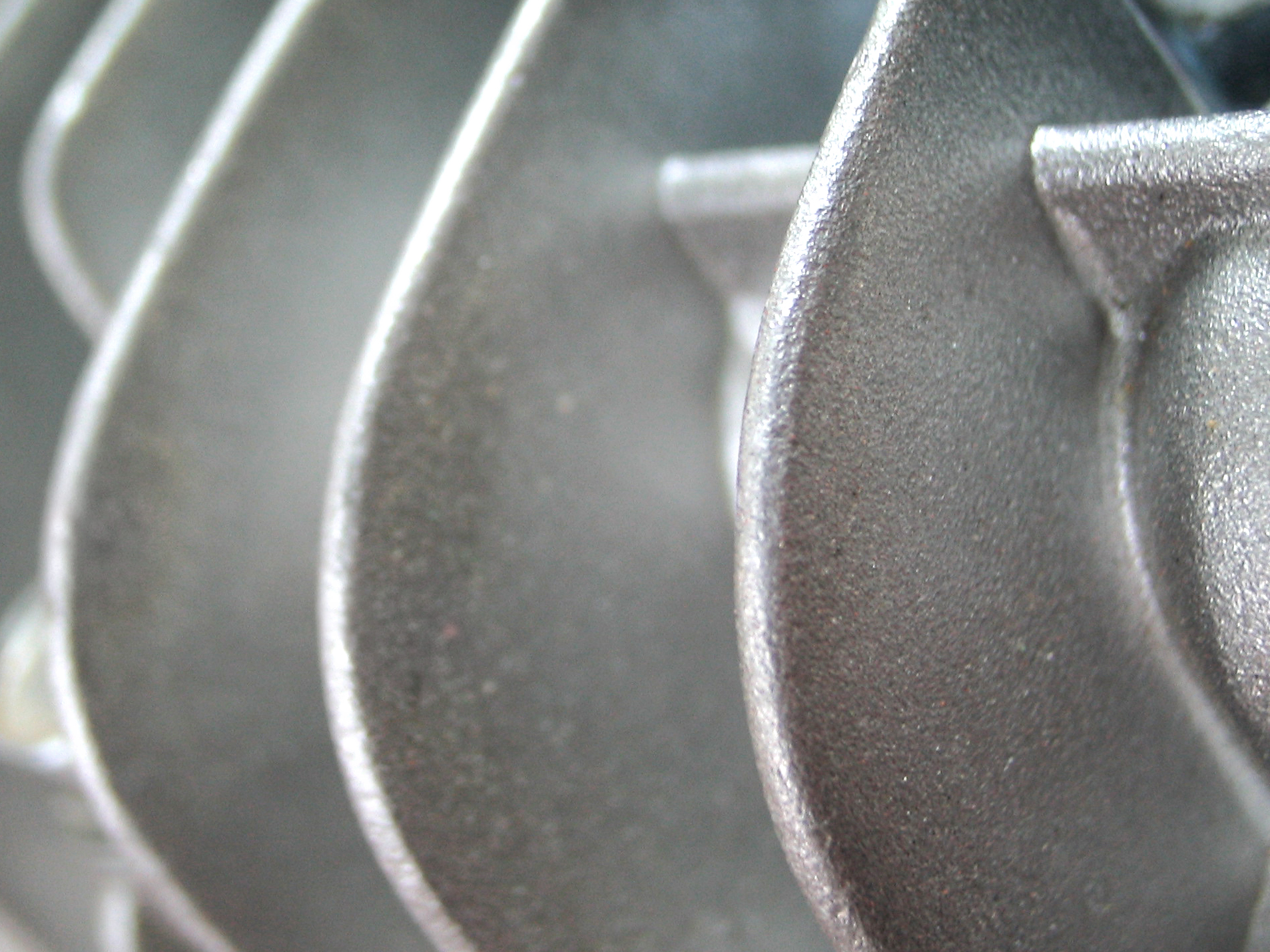## Application Range of Variety of Compressors and Compressor Selection

When I was involved in pre-FEED of well production and gas treatment, one of key deliverable is compressor studyIn this post, I want to share you about application range of variety of compressors (reciprocating, compressor, and axial-flow). In addition, I will also share the differences between those compressors.

### Application Range of Variety of Compressors

Figure below can estimate application range of variety of compressors. It is a function of inlet flow in actual cubic feet per minute and discharge pressure in psia.## Centrifugal Compressor Power Calculation

In this post I want to share you how to calculate centrifugal compressor power since there are so many process engineers are looking for it (and I also don’t know how).

To calculate centrifugal compressor power, we need these gas properties: compressibility factor (Z), molecular weight (MW), inlet gas temperature, inlet gas pressure, outlet gas pressure, adiabatic component (Cp/Cv), and mass flow rate.

Let’s start with an example while I show you step-by-step of the calculation method. In this example, we have these gas properties (I use US units).

Compressibility factor (Z) = 0.9972

Molecular weight (MW) = 18.15

Gas constant (R) = 1544/molecular weight

Inlet temperature (T1)  = 546 deg R

Inlet pressure (P1) = 15 psia (don’t forget to use absolute pressure)

Outlet pressure (P2) = 60 psia

K (Cp/Cv) = 1,274

Flow (W) = 0,184 lb/min